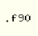# Numbers with a Gaussian distribution

(diff) ← Older revision | Latest revision (diff) | Newer revision → (diff)This page contains computer source code. If you intend to compile and use this code you must check for yourself the validity of the code. Please read the SklogWiki disclaimer.

Random number generators usually provide numbers with a uniform (flat) distribution. Sometimes it is desirable to generate numbers with some other distributions. The Gaussian (normal) distribution is of paramount imporance.

## Fortran 90 implementation

This Fortran 90 implementation is adapted from Ref. 1, based on an algorithm from the Numerical Recipes collection, Ref. 2. The function ran() calls a randon number generator:

```! Returns random numbers distributed following a Gaussian with
! unit variance

function gauss()

implicit none

real gauss

real v1,v2,r

real ranmar

do
v1=2.0*ranmar()-1.0
v2=2.0*ranmar()-1.0
r=v1*v1+v2*v2
if(r.lt.1.0) exit
enddo

gauss=v1*sqrt(-2.0*log(r)/r)

return

end function gauss
```